# please answer asap V1 = 8 (V) R1 = 8 (12) V2 = 26 (V) Problem...please answer asap

V1 = 8 (V) R1 = 8 (12) V2 = 26 (V) Problem 1 Using Kirchhoff's rules in the circuit of image, V4 = 28 (V) V3 = 20 (V) A- Calculate the current in the circuit. B- Show the direction current in the circuit. C- Calculate the voltage across resistor R1 D- Calculate the power delivered to R2 E- After 15 minutes of working of circuit, how much energy is delivered to resistor Rı? R2 = 7 (12)

This Homework Help Question: "please answer asap V1 = 8 (V) R1 = 8 (12) V2 = 26 (V) Problem..." No answers yet.

We need 10 more requests to produce the answer to this homework help question. Share with your friends to get the answer faster!

0 /10 have requested the answer to this homework help question.

Once 10 people have made a request, the answer to this question will be available in 1-2 days.
All students who have requested the answer will be notified once they are available.

#### Earn Coin

Coins can be redeemed for fabulous gifts.

Similar Homework Help Questions
• ### V1 = 8 (V) R1 = 8 (2) V2 = 26 (V) HI Problem 1 Using...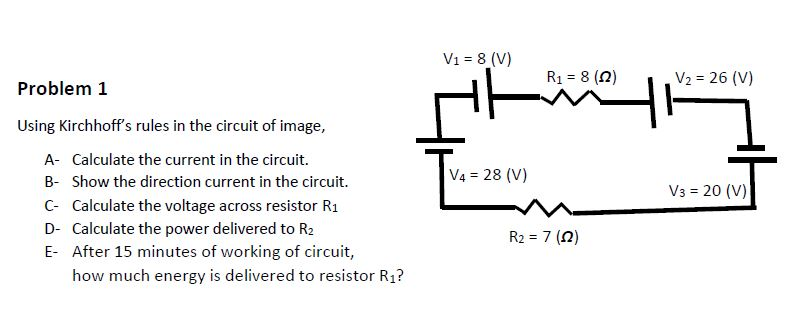V1 = 8 (V) R1 = 8 (2) V2 = 26 (V) HI Problem 1 Using Kirchhoff's rules in the circuit of image, A- Calculate the current in the circuit. B- Show the direction current in the circuit. C- Calculate the voltage across resistor R1 D- Calculate the power delivered to R2 E- After 15 minutes of working of circuit, how much energy is delivered to resistor Rı? V4 = 28 (V) V3 = 20 (V) R2 = 7 (12)

• ### V1 = 8 (V) R1 = 8 (0) V2 = 26 (V) Problem 1 Using Kirchhoff's...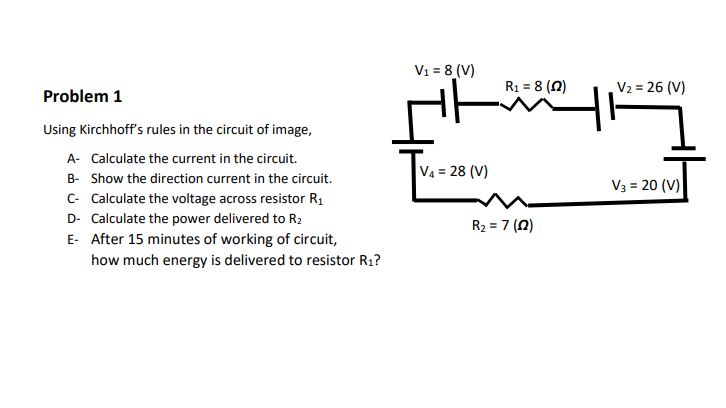V1 = 8 (V) R1 = 8 (0) V2 = 26 (V) Problem 1 Using Kirchhoff's rules in the circuit of image, A- Calculate the current in the circuit. B- Show the direction current in the circuit. C- Calculate the voltage across resistor R1 D- Calculate the power delivered to R2 E- After 15 minutes of working of circuit, how much energy is delivered to resistor Rı? V4 = 28 (V) V3 = 20 (V) R2 = 7 (2)

• ### V1 = 8 (V) Problem 1 R1 = 8 (0) V2 = 26 (V) VA =...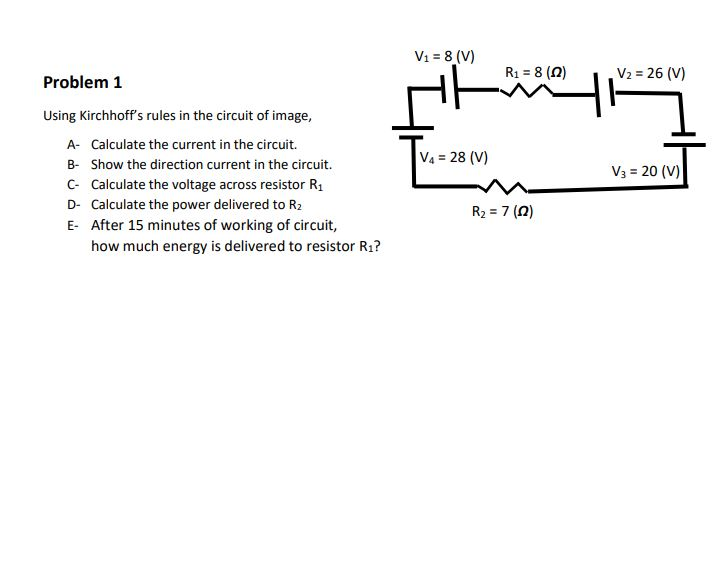V1 = 8 (V) Problem 1 R1 = 8 (0) V2 = 26 (V) VA = 28 (V) V3 = 20 (V) Using Kirchhoff's rules in the circuit of image, A- Calculate the current in the circuit. B- Show the direction current in the circuit. C- Calculate the voltage across resistor R1 D- Calculate the power delivered to R2 E After 15 minutes of working of circuit, how much energy is delivered to resistor Rı? R2 = 7(0)

• ### V1 = 8 (V) R1 = 8 (0) V2 = 26 (V) Problem 1 Using Kirchhoff's...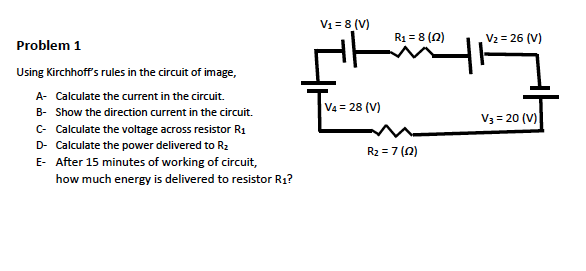V1 = 8 (V) R1 = 8 (0) V2 = 26 (V) Problem 1 Using Kirchhoff's rules in the circuit of image, A- Calculate the current in the circuit. B- Show the direction current in the circuit. C- Calculate the voltage across resistor Ra D- Calculate the power delivered to Rz E- After 15 minutes of working of circuit, how much energy is delivered to resistor R1? V4 = 28 (V) V3 = 20 (V) R2 = 7(0

• ### V1 = 8 (V) Problem 1 R1 = 8 (0) V2 = 26 (V) H V4...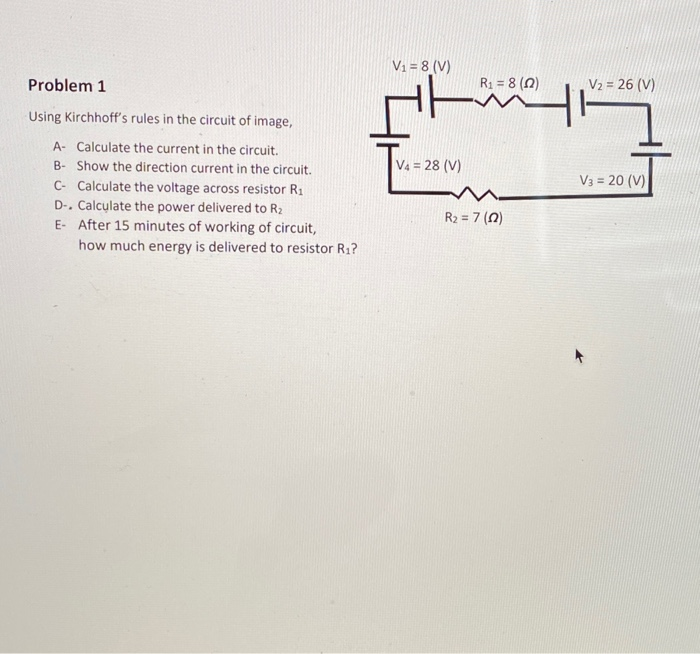V1 = 8 (V) Problem 1 R1 = 8 (0) V2 = 26 (V) H V4 = 28 (V) Using Kirchhoff's rules in the circuit of image, A- Calculate the current in the circuit. B- Show the direction current in the circuit. C- Calculate the voltage across resistor Ri D-. Calculate the power delivered to R2 E- After 15 minutes of working of circuit, how much energy is delivered to resistor R.? V3 = 20 (V) R2 = 7(0)

• ### please do all parts a,b,c,d,and e V1 = 8 (V) R1 = 8 (2) V2 =...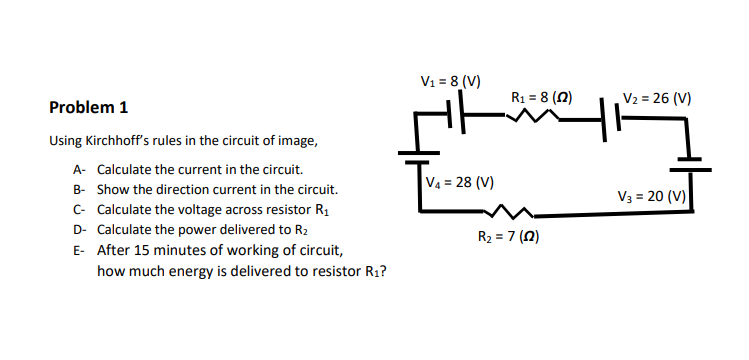please do all parts a,b,c,d,and e V1 = 8 (V) R1 = 8 (2) V2 = 26 (V) 7 Problem 1 Using Kirchhoff's rules in the circuit of image, A- Calculate the current in the circuit. B- Show the direction current in the circuit. C- Calculate the voltage across resistor R1 D- Calculate the power delivered to R2 E- After 15 minutes of working of circuit, how much energy is delivered to resistor Rı? V4 = 28 (V) V3 =...

• ### V1 = 8 (V) R1 = 8 (2) V2 = 26 (V) 두 - Problem 1...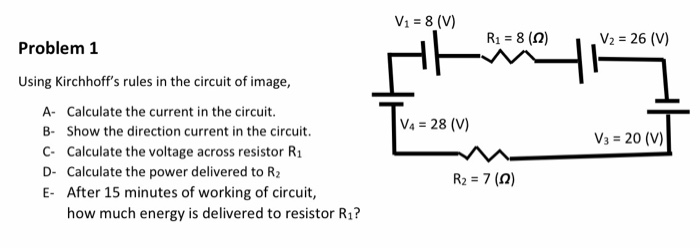V1 = 8 (V) R1 = 8 (2) V2 = 26 (V) 두 - Problem 1 Using Kirchhoff's rules in the circuit of image, A- Calculate the current in the circuit. B- Show the direction current in the circuit. C. Calculate the voltage across resistor R1 D- Calculate the power delivered to R2 E- After 15 minutes of working of circuit, how much energy is delivered to resistor Rı? |VA = 28 (V) Va = 20 (V) R2 = 7...

• ### Please show any equation manipulation. Vi = 8 (V) Problem 1 R1 = 8 (1) V2...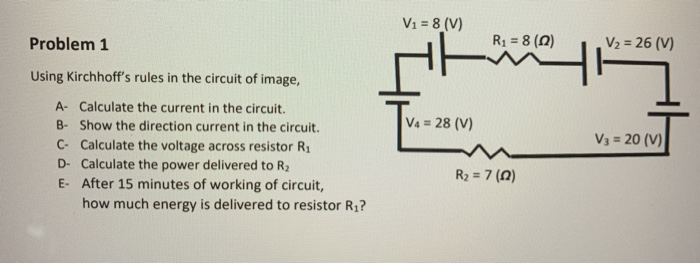Please show any equation manipulation. Vi = 8 (V) Problem 1 R1 = 8 (1) V2 = 26 (V) HH V4 = 28 (V) Using Kirchhoff's rules in the circuit of image, A- Calculate the current in the circuit. B- Show the direction current in the circuit. C- Calculate the voltage across resistor R. D- Calculate the power delivered to R2 E- After 15 minutes of working of circuit, how much energy is delivered to resistor Rı? V3 = 20...

• ### V2 = 8 (V) Ry = 8 (0) V2 = 26 (V) Problem 1 Using Kirchhoff's...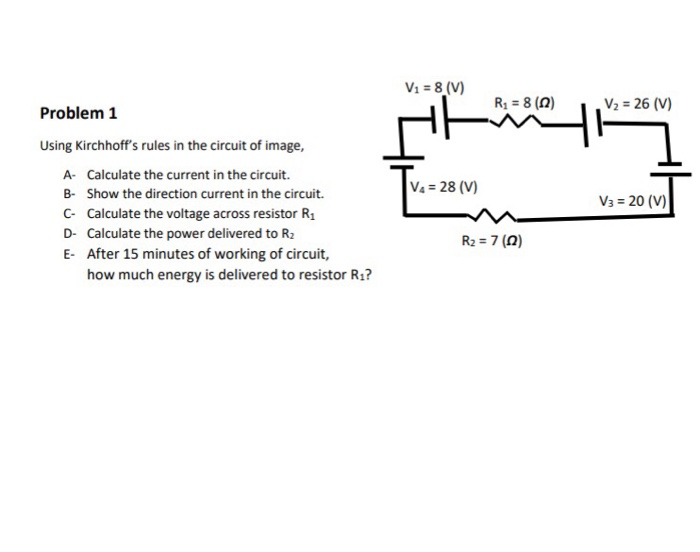V2 = 8 (V) Ry = 8 (0) V2 = 26 (V) Problem 1 Using Kirchhoff's rules in the circuit of image, A- Calculate the current in the circuit. B- Show the direction current in the circuit. C- Calculate the voltage across resistor R1 D- Calculate the power delivered to R2 E- After 15 minutes of working of circuit, how much energy is delivered to resistor Rı? T-28 V2 = 28 (V) V3 = 20 (7) R2 = 7(0)

• ### need before 12:00 noon central time july 30, 2020 V1 = 8 (V) R1 = 8...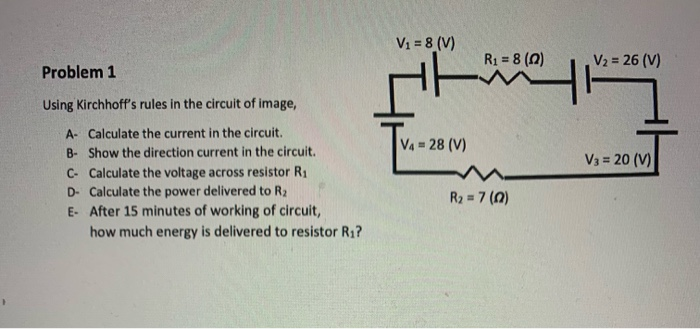need before 12:00 noon central time july 30, 2020 V1 = 8 (V) R1 = 8 (0) V2 = 26 (V) Problem 1 Using Kirchhoff's rules in the circuit of image, V4 = 28 (V) V3 = 20 (1) A- Calculate the current in the circuit. B- Show the direction current in the circuit. C- Calculate the voltage across resistor Ri D- Calculate the power delivered to R2 E- After 15 minutes of working of circuit, how much energy is...